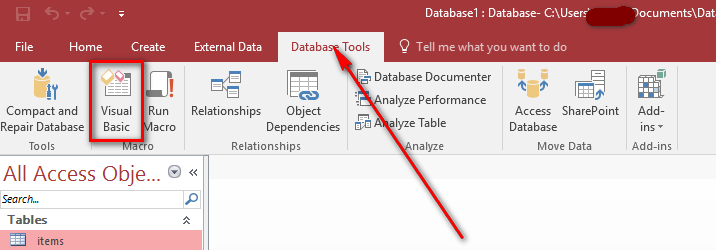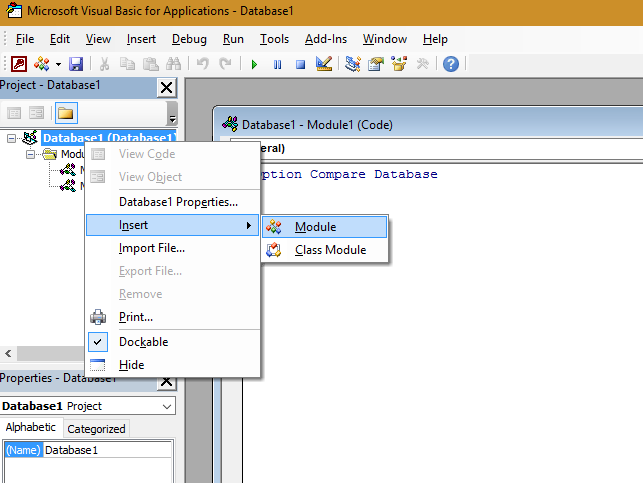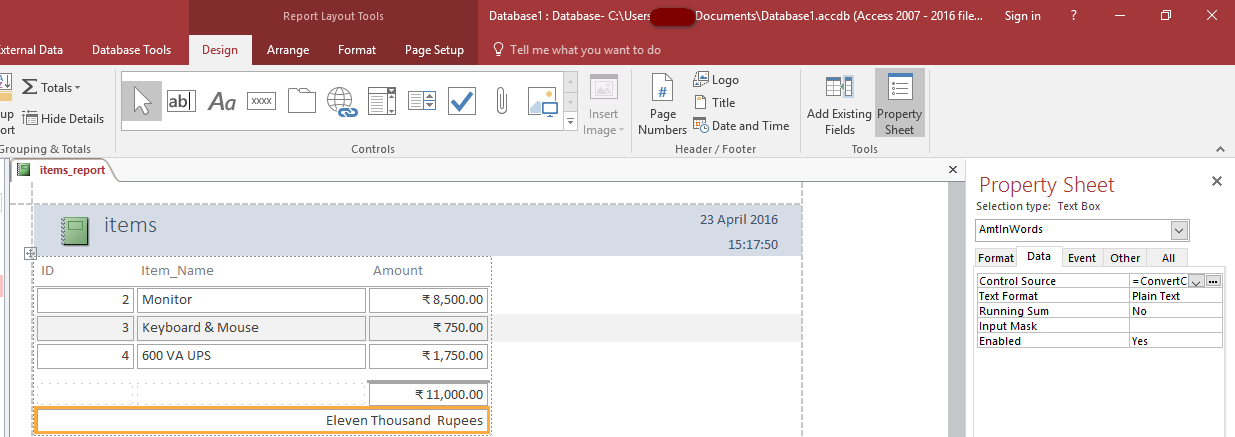# Convert Currency in Number to Words - MS Access

We may require currency in both number & words in MS Access Reports. Here I am giving a easiest way to implement this. This artice is extension of my previous blog post Convert Currency in Number to Words (Indian Rupees)

Note : Screenshots may differ for your Access Version, If you have any queries, comment below.

1. Go to Database Tools Tab in MS Access, click on Visual Basic2. Right click on Modules, select Insert -> Module3. Copy and paste the VBA code given below.

``````
Function ConvertCurrencyToEnglish(ByVal MyNumber)
' Edited by Karthikeyan [email protected]
Dim Temp
Dim Rupees, Paise
Dim DecimalPlace, Count

ReDim Place(9) As String
Place(2) = " Thousand "
Place(3) = " lakh "
Place(4) = " Crore "

' Convert MyNumber to a string, trimming extra spaces.
MyNumber = Trim(Str(MyNumber))

' Find decimal place.
DecimalPlace = InStr(MyNumber, ".")

' If we find decimal place...
If DecimalPlace > 0 Then
' Convert Paise
Temp = Left(Mid(MyNumber, DecimalPlace + 1) & "00", 2)
' Hi! Note the above line Mid function it gives right portion
' after the decimal point
'if only . and no numbers such as 789. accures, mid returns nothing
' to avoid error we added 00
' Left function gives only left portion of the string with specified places here 2

Paise = ConvertTens(Temp)

' Strip off paise from remainder to convert.
MyNumber = Trim(Left(MyNumber, DecimalPlace - 1))
End If

Count = 1
If MyNumber <> "" Then

' Convert last 3 digits of MyNumber to Indian Rupees.
Temp = ConvertHundreds(Right(MyNumber, 3))

If Temp <> "" Then Rupees = Temp & Place(Count) & Rupees

If Len(MyNumber) > 3 Then
' Remove last 3 converted digits from MyNumber.
MyNumber = Left(MyNumber, Len(MyNumber) - 3)
Else
MyNumber = ""
End If

End If

' convert last two digits to of mynumber
Count = 2

Do While MyNumber <> ""
Temp = ConvertTens(Right("0" & MyNumber, 2))

If Temp <> "" Then Rupees = Temp & Place(Count) & Rupees
If Len(MyNumber) > 2 Then
' Remove last 2 converted digits from MyNumber.
MyNumber = Left(MyNumber, Len(MyNumber) - 2)

Else
MyNumber = ""
End If
Count = Count + 1

Loop

' Clean up rupees.
Select Case Rupees
Case ""
Rupees = ""
Case "One"
Rupees = "Rupee One"
Case Else
Rupees = "Rupees " & Rupees
End Select

' Clean up paise.
Select Case Paise
Case ""
Paise = ""
Case "One"
Paise = "One Paise"
Case Else
Paise = Paise & " Paise"
End Select

If Rupees = "" Then
ConvertCurrencyToEnglish = Paise & " Only"
ElseIf Paise = "" Then
ConvertCurrencyToEnglish = Rupees & " Only"
Else
ConvertCurrencyToEnglish = Rupees & " and " & Paise & " Only"
End If

End Function

Private Function ConvertDigit(ByVal MyDigit)
Select Case Val(MyDigit)
Case 1: ConvertDigit = "One"
Case 2: ConvertDigit = "Two"
Case 3: ConvertDigit = "Three"
Case 4: ConvertDigit = "Four"
Case 5: ConvertDigit = "Five"
Case 6: ConvertDigit = "Six"
Case 7: ConvertDigit = "Seven"
Case 8: ConvertDigit = "Eight"
Case 9: ConvertDigit = "Nine"
Case Else: ConvertDigit = ""
End Select

End Function

Private Function ConvertHundreds(ByVal MyNumber)
Dim Result As String

' Exit if there is nothing to convert.
If Val(MyNumber) = 0 Then Exit Function

' Append leading zeros to number.
MyNumber = Right("000" & MyNumber, 3)

' Do we have a hundreds place digit to convert?
If Left(MyNumber, 1) <> "0" Then
Result = ConvertDigit(Left(MyNumber, 1)) & " Hundred "
End If

' Do we have a tens place digit to convert?
If Mid(MyNumber, 2, 1) <> "0" Then
Result = Result & ConvertTens(Mid(MyNumber, 2))
Else
' If not, then convert the ones place digit.
Result = Result & ConvertDigit(Mid(MyNumber, 3))
End If

ConvertHundreds = Trim(Result)
End Function

Private Function ConvertTens(ByVal MyTens)
Dim Result As String

' Is value between 10 and 19?
If Val(Left(MyTens, 1)) = 1 Then
Select Case Val(MyTens)
Case 10: Result = "Ten"
Case 11: Result = "Eleven"
Case 12: Result = "Twelve"
Case 13: Result = "Thirteen"
Case 14: Result = "Fourteen"
Case 15: Result = "Fifteen"
Case 16: Result = "Sixteen"
Case 17: Result = "Seventeen"
Case 18: Result = "Eighteen"
Case 19: Result = "Nineteen"
Case Else
End Select
Else
' .. otherwise it's between 20 and 99.
Select Case Val(Left(MyTens, 1))
Case 2: Result = "Twenty "
Case 3: Result = "Thirty "
Case 4: Result = "Forty "
Case 5: Result = "Fifty "
Case 6: Result = "Sixty "
Case 7: Result = "Seventy "
Case 8: Result = "Eighty "
Case 9: Result = "Ninety "
Case Else
End Select

' Convert ones place digit.
Result = Result & ConvertDigit(Right(MyTens, 1))
End If

ConvertTens = Result
End Function
``````

4. Save and Close the Visual Application Window.

5. Create a table, and report of your choice.

6. Add a Text a field in report where you want to convert the number to words, set Control Source propery to the currency conversion formula.

For example in my report sum of amount field need to be converted to words.

I have added a text field named AmtInWords and set the control source property value to the following formula.

=ConvertCurrencyToEnglish(Sum([Amount]))### Video Demo

Update 02/03/2017 : 'Rupee/ Rupees' word added as prefix, 'Only' word added as suffix.

Category

Isyaku Bala

Jan 29, 2021 - 17:50

Sorry for the previous post, Kindly assist on how i can format the Code in Rupees for the convert of number to word in access to a local currency

Karthikeyan

Jan 02, 2021 - 05:57

Set the initial value of the field to zero.

Md Sajal Sikder

Jun 08, 2020 - 15:53

thank you

Md Sajal Sikder

Jun 08, 2020 - 13:39

Divya

Apr 30, 2020 - 21:37

This is working on Excel 2010 also in Office 365

sridharan

Jul 29, 2018 - 18:49

plz share code and guide me how to use

sridharan

Jul 29, 2018 - 18:48

it is not working in my excel 2010

Karthikeyan

Jun 08, 2018 - 14:55

In reply to by Ramanand bairwa

Is number displaying correctly without using currencyconverter?

Ramanand bairwa

Jun 08, 2018 - 10:53

I pasted vb code as from Download Sample access database, but in Access Text Box numbers are not converting in words and displays : #Name?, I wrote in text box of Access : ConvertCurrencyToEnglish(Int([BP]/2+0.05)). Please suggest me. What went wrong with this work?

firoz khan

Dec 14, 2017 - 11:06

Sir,
I have copy your vba code but I have seen #Name ware I have set Formula

SARAVANAN VENNILA

Nov 26, 2017 - 07:48

TANK

Rajagopal P

Oct 12, 2017 - 13:45

Thank you

Karthikeyan

Sep 08, 2017 - 18:10

Please, Post screenshot or attach the file.

Manoj

Sep 07, 2017 - 15:29

IT is showing #Name?

Syed Md. Tousif

Apr 21, 2017 - 21:11

Got it.......i find my goal....thanx karthikeyan

Karthikeyan

Apr 21, 2017 - 21:05

In reply to by Syed Md. Tousif

Try copying the module1 from the sample access file and paste it into your access file.

Syed Md. Tousif

Apr 21, 2017 - 20:57

It worked only for your attached file,....in my file it not worked....
IT is showing #Name?

Syed Md. Tousif

Apr 21, 2017 - 20:40

Your attached file also saying #Error

Karthikeyan

Apr 14, 2017 - 20:19

There may be an issue with the code you saved in module.
If not working, attach the file with sample data.

zeema

Apr 13, 2017 - 12:22

i have copied the code
and saved
and set the control source property value to the following formula.

=ConvertCurrencyToEnglish(Sum([amount]))
mine field name is amount so i have given amount in formula

but i m getting #Error when i execute

WhiteLotus1

Mar 04, 2017 - 16:37

Thank you Sir.
It now shows 'Rupees' before the number conversion .....

Mar 02, 2017 - 18:59

Hi,
Thanks.

WhiteLotus1

Feb 22, 2017 - 13:19

Thank you. I changed Expression, now it shows two Rupees :)

Output
Rupees Hundred Rupees Only

another problem is that i have to convert number to words for both INR AND USD.

Karthikeyan

Feb 22, 2017 - 13:15

Yes, place Rupees before the expression. ="Rupees " &ConvertCurrencyToEnglish([MyTextBoxName])) & " Only"

WhiteLotus1

Feb 22, 2017 - 13:06

Thank you for sharing code.
Is it possible to write Rupees *Before* the currency is converted into words
e.g. Rupees Hundred Only
Currently it shows Hundred Rupees Only

I have added " Only" to the expression builder
i.e. =ConvertCurrencyToEnglish([MyTextBoxName])) & " Only"

The reason for this request is that after money roundoff act, In Invoice or PO grandtotal, amount is always round off. hence conversion of paisa to words is not needed.

Thank you.